# A Nonlinear Programming Model with Multiple Constraints

[Page 461]

Now that we have shown how Excel can be used to solve nonlinear problems, we can look at more complex problemsfor example, a problem with more than one constraint. Consider the Western Clothing Company example presented earlier, except now the company produces two kinds of jeans, designer and straight-leg, and production is subject to resource constraints for denim cloth, cutting time, and sewing time. The company sells its jeans to several upscale clothing store chains, and sales demand is dependent on the price at which the company sells the jeans. The demand for designer jeans ( x 1 ) and the demand for straight-leg jeans ( x 2 ) are defined by the following relationships:

x 1 = 1,500 24.6 p 1

x 2 = 2,700 63.8 p 2

where

p 1 = price of designer jeans

p 2 = price of straight-leg jeans

The cost of producing designer jeans is \$12 per pair, and the cost of producing straight-leg jeans is \$9 per pair; thus, the objective function for profit is

maximize Z = ( p 1 12) x 1 + ( p 2 9) x 2

The production of jeans is subject to the following resource constraints for available cloth, cutting time, and sewing time: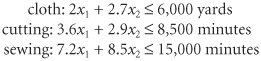The complete nonlinear programming model formulation is summarized as follows :

maximize Z = ( p 1 12) x 1 + ( p 2 9) x 2

subject to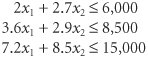where

x 1 = 1,500 24.6 p 1

x 2 = 2,700 63.8 p 2

p 1 = price of designer jeans

p 2 = price of straight-leg jeans

Notice that the decision variables for this problem are p 1 and p 2 , not x 1 and x 2 . The demand variables, x 1 and x 2 , are functions of price and, thus, are dependent variables. Also notice that we did not substitute the functional relationships for x 1 and x 2 into the objective function, creating a quadratic function. The model is still nonlinear, but we can solve it directly with Excel in its present form.

Exhibit 10.9 shows an Excel spreadsheet set up to solve our Western Clothing Company example with constraints. The decision variables for price are contained in cells D5:D6 . The formula for demand for designer jeans is contained in cell C5 and is shown on the formula bar at the top of the screen. The formula for total profit, =SUMPRODUCT ( C5:C6,E5:E6 ), is contained in cell E7. The formulas for the resource constraints are contained in cells C11, C12, and C13, and the resource availabilities for each constraint are in cells D11:D13 . The Solver Parameters window and solution are shown in Exhibit 10.10 and Exhibit 10.11, respectively.

[Page 462]
##### Exhibit 10.9.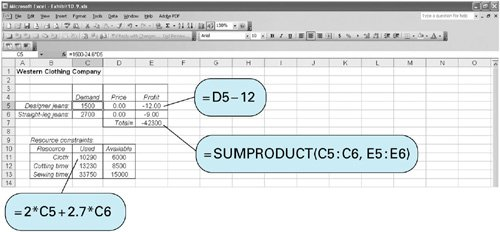##### Exhibit 10.10.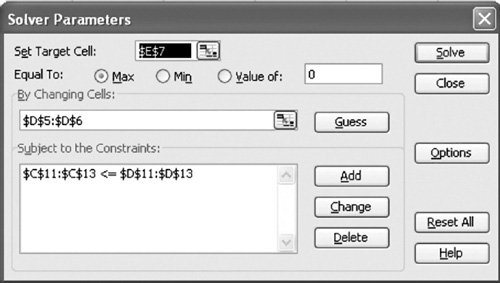##### Exhibit 10.11.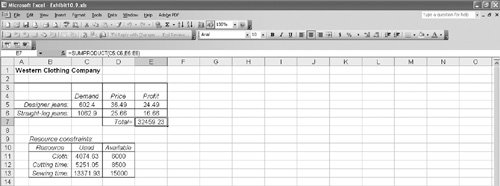Introduction to Management Science (10th Edition)
ISBN: 0136064361
EAN: 2147483647
Year: 2006
Pages: 358

Similar book on Amazon

flylib.com © 2008-2017.
If you may any questions please contact us: flylib@qtcs.net